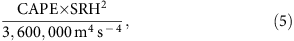## Introduction

Hazardous convective weather (tornadoes, hail and damaging wind) associated with severe thunderstorms affects large portions of the United States. Tornadoes cause particularly intense damage. Over a recent 10-year period (2005–2014), tornadoes in the United States resulted in an average of 110 deaths per year and annual losses ranging from $500 million to$9.6 billion1. The largest societal impacts from tornadoes are from ‘outbreaks’ in which multiple tornadoes occur in a single weather event. Tornado outbreaks across the eastern two-thirds of the United States were associated with 79% of all tornado fatalities over the period 1972–2010 (ref. 2) and are routinely responsible for billion-dollar loss events3.

## Results

### Data and sensitivity analyses

We use data from 1954 to 2014, which are generally considered reliable. Because of concerns regarding the data before 1977 (‘Methods’ section, Supplementary Fig. 1), we repeat some analysis using the more recent period 1977–2014 to test the robustness of the results (Supplementary Figs 2–5). We exclude the weakest tornadoes from our analysis and denote the remaining tornadoes as F1+ tornadoes (‘Methods’ section). We repeat some of the analysis restricted to more intense tornadoes (F2+; Supplementary Figs 7–10).

### Number of tornadoes per outbreakFigure 1: Time series of counts and clustering of F1+ tornadoes 1954–2014 in the contiguous US.

The fact that the variance is increasing several times faster than the mean is especially noteworthy: it indicates a changing distribution in which the likelihood of extreme outbreaks is increasing faster than what the trend in mean alone would suggest. The coefficient of dispersion of a probability distribution with a positive mean is the ratio of its variance to its mean. Values greater than one (over-dispersion) indicate more clustering than a Poisson variable. For instance, European windstorms exhibit over-dispersion and serial clustering that increases with intensity21 with implications for the return intervals of rare events22. Taylor’s law (TL) relates the mean and variance of a probability distribution by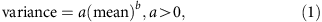where a and b are constants15,16. A value of b>1 indicates that the coefficient of dispersion increases with the mean. The annual mean and annual variance of the number of tornadoes per outbreak approximately satisfy TL with b=4.3±0.44 and log a=−6.74±1.12 (Fig. 2d); consistent values are seen over the period 1977–2014 (Supplementary Fig. 3d). (Throughout log is the natural logarithm.) The value of b here is remarkable since in most ecological applications, the TL exponent seldom exceeds 2. The TL exponent can be greater than 2 for lognormal distributions with changing parameters (Supplementary Discussion and Supplementary Fig. 11). The TL scaling of tornado outbreak severity reveals a remarkably regular relation between annual mean and annual variance that extends over the full range of the data, even for years like 2011 which are extreme in mean and variance. The data from 1974 deviate most from TL scaling, with the excessive variance reflecting the 3–4 April ‘Super Outbreak.’

The upward trend in the number of tornadoes per outbreak provides an interpretation for the observed TL scaling since TL scaling arises in models of stochastic multiplicative growth17. In such models, the quantity N(t+1) at time t+1 is related to its previous value N(t) by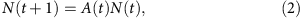where A(t) is the random multiplicative factor by which N(t) grows or declines from one time to the next. Here N(t) is the annual average number of tornadoes per outbreak, and each integer value of t represents one calendar year. The Lewontin–Cohen (LC) model for stochastic multiplicative growth assumes that the A(t) are independently and identically distributed for all t≥0 with finite mean M>0 and finite variance V . If M≠1, N(t) follows TL asymptotically with17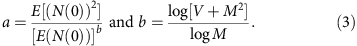Here we estimate (‘Methods’ section) M=1.03 and V=0.068, which leads to TL parameters b=3.98 and log(a)= −5.84. Both values are consistent with the least-squares (LS) estimates of the corresponding parameters of TL (Fig. 2d). The LS estimates are also consistent with the values from LC theory during 1977–2014 (Supplementary Fig. 3d). 95% confidence intervals for M and V show that the hypothesis of no growth (M=1) under which equation (3) is not valid cannot be rejected (Supplementary Table 1). The Supplementary Discussion provides additional description of how the LC model leads to TL scaling with exponent approximately 4.

### Fujita-kilometers per outbreak

Another measure of outbreak severity is Fujita-kilometers (ref. 2; F-km) which is the sum (over all tornadoes in an outbreak) of each tornado’s path length in kilometers multiplied by its Fujita or Enhanced Fujita rating (‘Methods’ section). Annual totals of outbreak F-km, mean number of F-km per outbreak and the variance of F-km per outbreak do not show significant trends over the period 1954–2014 (Fig. 3a–c). The mean number of F-km per outbreak and the variance of F-km per outbreak show marginally significant trends over the recent period 1977–2014 (Supplementary Fig. 4a–c). The TL parameters relating the mean and variance of F-km per outbreak are b=2.77±0.30 and log a=−3.75±1.71 (Fig. 3d). The lack of robust trends means that LC theory is not appropriate to explain the TL scaling of F-km. However, TL scaling also arises from the sampling of stationary skewed distributions20. For a distribution with mean m, variance v, skewness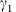and coefficient of variation CV, theory20 predicts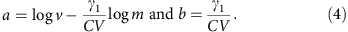Here, excluding two outlier outbreaks from the calculation of the distribution parameters (‘Methods’ section, Supplementary Fig. 5), equation (4) gives b=2.71 and log a=−3.05, both of which are consistent with the LS estimates of the TL parameters for F-km per outbreak. (We use ‘outlier’ to indicate values far from other observations, not to suggest that the unusual values are the result of measurement error.) Therefore TL scaling of F-km per outbreak could be explained by sampling variability.

### Sensitivity to outbreak definition

Another concern is that the results are sensitive to the details of the outbreak definition. We assess the robustness of the results to the E/F1 threshold by repeating the analysis with tornadoes rated E/F2 and higher, denoted F2+ (Supplementary Figs 7–10). We use the period 1977–2014 because the annual number of F2+ tornadoes display a substantial decrease (not shown) around the 1970s that is likely related to the introduction of the F-scale. Overall the F2+ results are remarkably similar to the F1+ ones. The annual number of F2+ tornadoes has an insignificantly negative trend during 1977–2014 (Supplementary Fig. 7a), and the percentage of F2+ tornadoes occurring in F2+ outbreaks has a significant positive trend (Supplementary Fig. 7b). Although the number of F2+ outbreaks shows no significant trend, the mean number of tornadoes per F2+ outbreak and its variance both have significant upward trends (Supplementary Fig. 8a–c). The TL exponent for number of tornadoes per F2+ outbreak is 3.65 and is consistent with LC theory (Supplementary Fig. 8d). Annual totals of F2+ outbreak F-km have no significant trend (Supplementary Fig. 9a). Mean F-km per F2+ outbreak does have a significant upward trend, but variance does not (Supplementary Fig. 9b,c). The TL scaling of F2+ outbreak F-km (Supplementary Fig. 9d) is consistent with sampling variability when 2011 is excluded (Supplementary Fig. 10).

## Discussion

These findings have important implications for tornado risk in the United States and perhaps elsewhere, though we have examined only the US data. First, the number of tornadoes per outbreak is increasing. However, there is less evidence that F-km per outbreak are increasing. Both the number of tornadoes per outbreak and F-km per outbreak follow TL, which relates mean and variance. We find that TL scaling for the number of tornadoes per outbreak is compatible with multiplicative growth, and that TL scaling for F-km per outbreak could be due to sampling variability. Finally, the key implication of TL scaling is that both number of tornadoes per outbreak and F-km per outbreak exhibit extreme over-dispersion which increases with mean. When the average tornado outbreak severity gets worse, the high extreme of severity rises even faster and the low extreme falls even faster, by either measure of severity.

## Methods

### Outbreak data

The same outbreak calculation procedure is repeated but considering only reports of tornadoes rated F/EF2 or greater, denoted as F2+. These outbreak events are referred to as F2+ outbreaks (Supplementary Figs 7–10). Only F2+ tornadoes are used to calculate tornado numbers and F-km of F2+ outbreaks.

### Trends

All trends and 95% confidence intervals are assessed using linear regression and ordinary least squares, assuming approximately normal distributions of residuals. The growth rates of the annual mean and variance of the outbreak severity measures in Fig. 2b,c, Fig. 3b,c and Supplementary Figs 3b,c; 4b,c; 8b,c and 9b,c are computed by assuming exponential growth and fitting a linear trend to the logarithms of the data. All the other trends are fitted using untransformed data.

### TL parameters

The TL parameters in Figs 2d, 3d and 4d and Supplementary Figs 3d, 4d, 8d and 9d and 95% confidence intervals are estimated using ordinary least-squares regression with the logarithms of the mean and variance.

### TL parameters implied by LC theory

The growth factor in the LC model is computed from A(t)=N(t+1)/N(t), where N(t) is the average number of tornadoes per outbreak in calendar year t. The 95% confidence intervals for the mean and variance of A(t) are computed from 10,000 bootstrap samples and reported in Supplementary Table 1. The mean and variance of A(t) are used to compute a prediction of the slope b using equation (3). The TL parameter a is estimated from equation (1) evaluated at the initial year, either 1954 or 1977.

### TL parameters implied by sampling variability

There are 1,361 cases of outbreak F-km, and their distribution is highly right-skewed (Supplementary Figs 5a and 10a). The F-km values for the 1974 Super Outbreak and the 25–28 April 2011 tornado outbreak are more than 26 standard deviations above the mean of the data on an arithmetic scale and more than six standard deviations above the mean of the log-transformed data, when means and standard deviations are calculated after withholding the two extreme values. These outliers (values that are far from other observations) have a substantial impact on the estimates of the mean, variance and skewness of the F-km distribution. Despite their rarity, about 86% (1−(1−2/1,361)1361) of the bootstrap samples will contain one or both of these two events. The presence of the outliers results in bimodal distributions of the TL slope and intercept estimates (Supplementary Figs 5b,c and 10b,c) computed from equation (4), depending on whether or not the outlier values are in the particular bootstrap sample. Removal of the outliers results in unimodal distributions, whose ranges are consistent with the least-squares estimates of the TL slope and intercept for F-km (Supplementary Figs 5d,e and 10d,e).

### Environmental proxies